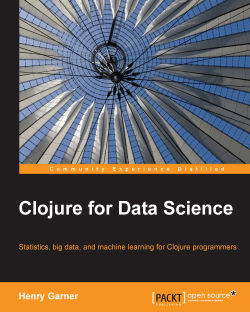# Clojure for Data Science5 (4 reviews total)
By Henry Garner
• \$41.99 eBook
• \$51.99 Print + eBook
• \$12.99 eBook + Subscription
What do you get with a Packt Subscription?

• Constantly updated with 100+ new titles each month
• Breadth and depth in over 1,000+ technologies

The term “data science” has been widely used to define this new profession that is expected to interpret vast datasets and translate them to improved decision-making and performance. Clojure is a powerful language that combines the interactivity of a scripting language with the speed of a compiled language. Together with its rich ecosystem of native libraries and an extremely simple and consistent functional approach to data manipulation, which maps closely to mathematical formula, it is an ideal, practical, and flexible language to meet a data scientist’s diverse needs.

Taking you on a journey from simple summary statistics to sophisticated machine learning algorithms, this book shows how the Clojure programming language can be used to derive insights from data. Data scientists often forge a novel path, and you’ll see how to make use of Clojure’s Java interoperability capabilities to access libraries such as Mahout and Mllib for which Clojure wrappers don’t yet exist. Even seasoned Clojure developers will develop a deeper appreciation for their language’s flexibility!

You’ll learn how to apply statistical thinking to your own data and use Clojure to explore, analyze, and visualize it in a technically and statistically robust way. You can also use Incanter for local data processing and ClojureScript to present interactive visualisations and understand how distributed platforms such as Hadoop sand Spark’s MapReduce and GraphX’s BSP solve the challenges of data analysis at scale, and how to explain algorithms using those programming models.

Above all, by following the explanations in this book, you’ll learn not just how to be effective using the current state-of-the-art methods in data science, but why such methods work so that you can continue to be productive as the field evolves into the future.

Publication date:
September 2015
Publisher
Packt
Pages
608
ISBN
9781784397180

## Chapter 1. Statistics

 "The people who cast the votes decide nothing. The people who count the votes decide everything." --Joseph Stalin

Over the course of the following ten chapters of Clojure for Data Science, we'll attempt to discover a broadly linear path through the field of data science. In fact, we'll find as we go that the path is not quite so linear, and the attentive reader ought to notice many recurring themes along the way.

Descriptive statistics concern themselves with summarizing sequences of numbers and they'll appear, to some extent, in every chapter in this book. In this chapter, we'll build foundations for what's to come by implementing functions to calculate the mean, median, variance, and standard deviation of numerical sequences in Clojure. While doing so, we'll attempt to take the fear out of interpreting mathematical formulae.

As soon as we have more than one number to analyze it becomes meaningful to ask how those numbers are distributed. You've probably already heard expressions such as "long tail" and the "80/20 rule". They concern the spread of numbers throughout a range. We demonstrate the value of distributions in this chapter and introduce the most useful of them all: the normal distribution.

The study of distributions is aided immensely by visualization, and for this we'll use the Clojure library Incanter. We'll show how Incanter can be used to load, transform, and visualize real data. We'll compare the results of two national elections—the 2010 United Kingdom general election and the 2011 Russian presidential election—and see how even basic analysis can provide evidence of potentially fraudulent activity.

All of the book's sample code is available on Packt Publishing's website at http://www.packtpub.com/support or from GitHub at http://github.com/clojuredatascience. Each chapter's sample code is available in its own repository.

### Note

The sample code for Chapter 1, Statistics can be downloaded from https://github.com/clojuredatascience/ch1-statistics.

Executable examples are provided regularly throughout all chapters, either to demonstrate the effect of code that has been just been explained, or to demonstrate statistical principles that have been introduced. All example function names begin with `ex-` and are numbered sequentially throughout each chapter. So, the first runnable example of Chapter 1, Statistics is named `ex-1-1`, the second is named `ex-1-2`, and so on.

## Running the examples

Each example is a function in the `cljds.ch1.examples` namespace that can be run in two ways—either from the REPL or on the command line with Leiningen. If you'd like to run the examples in the REPL, you can execute:

```lein repl
```

on the command line. By default, the REPL will open in the `examples` namespace. Alternatively, to run a specific numbered example, you can execute:

```lein run –-example 1.1
```

or pass the single-letter equivalent:

```lein run –e 1.1
```

We only assume basic command-line familiarity throughout this book. The ability to run Leiningen and shell scripts is all that's required.

### Tip

If you become stuck at any point, refer to the book's wiki at http://wiki.clojuredatascience.com. The wiki will provide troubleshooting tips for known issues, including advice for running examples on a variety of platforms.

In fact, shell scripts are only used for fetching data from remote locations automatically. The book's wiki will also provide alternative instructions for those not wishing or unable to execute the shell scripts.

The dataset for this chapter has been made available by the Complex Systems Research Group at the Medical University of Vienna. The analysis we'll be performing closely mirrors their research to determine the signals of systematic election fraud in the national elections of countries around the world.

### Note

Throughout this book we'll be making use of numerous datasets. Where possible, we've included the data with the example code. Where this hasn't been possible—either because of the size of the data or due to licensing constraints—we've included a script to download the data instead.

Chapter 1, Statistics is just such a chapter. If you've cloned the chapter's code and intend to follow the examples, download the data now by executing the following on the command line from within the project's directory:

```script/download-data.sh
```

The script will download and decompress the sample data into the project's data directory.

### Tip

If you have any difficulty running the download script or would like to follow manual instructions instead, visit the book's wiki at http://wiki.clojuredatascience.com for assistance.

We'll begin investigating the data in the next section.

## Inspecting the data

Throughout this chapter, and for many other chapters in this book, we'll be using the Incanter library (http://incanter.org/) to load, manipulate, and display data.

Incanter is a modular suite of Clojure libraries that provides statistical computing and visualization capabilities. Modeled after the extremely popular R environment for data analysis, it brings together the power of Clojure, an interactive REPL, and a set of powerful abstractions for working with data.

Each module of Incanter focuses on a specific area of functionality. For example `incanter-stats` contains a suite of related functions for analyzing data and producing summary statistics, while `incanter-charts` provides a large number of visualization capabilities. `incanter-core` provides the most fundamental and generally useful functions for transforming data.

Each module can be included separately in your own code. For access to stats, charts, and Excel features, you could include the following in your `project.clj`:

```  :dependencies [[incanter/incanter-core "1.5.5"]
[incanter/incanter-stats "1.5.5"]
[incanter/incanter-charts "1.5.5"]
[incanter/incanter-excel "1.5.5"]
...]```

If you don't mind including more libraries than you need, you can simply include the full Incanter distribution instead:

```:dependencies [[incanter/incanter "1.5.5"]
...]```

At Incanter's core is the concept of a dataset—a structure of rows and columns. If you have experience with relational databases, you can think of a dataset as a table. Each column in a dataset is named, and each row in the dataset has the same number of columns as every other. There are a several ways to load data into an Incanter dataset, and which we use will depend how our data is stored:

• If our data is a text file (a CSV or tab-delimited file), we can use the `read-dataset` function from `incanter-io`

• If our data is an Excel file (for example, an `.xls` or `.xlsx` file), we can use the `read-xls` function from `incanter-excel`

• For any other data source (an external database, website, and so on), as long as we can get our data into a Clojure data structure we can create a dataset with the `dataset` function in `incanter-core`

This chapter makes use of Excel data sources, so we'll be using `read-xls`. The function takes one required argument—the file to load—and an optional keyword argument specifying the sheet number or name. All of our examples have only one sheet, so we'll just provide the file argument as string:

```(ns cljds.ch1.data
(:require [clojure.java.io :as io]
[incanter.core :as i]
[incanter.excel :as xls]))```

In general, we will not reproduce the namespace declarations from the example code. This is both for brevity and because the required namespaces can usually be inferred by the symbol used to reference them. For example, throughout this book we will always refer to `clojure.java.io` as `io`, `incanter.core` as `I`, and `incanter.excel` as `xls` wherever they are used.

We'll be loading several data sources throughout this chapter, so we've created a multimethod called `load-data` in the `cljds.ch1.data` namespace:

```(defmulti load-data identity)

(-> (io/resource "UK2010.xls")
(str)

In the preceding code, we define the `load-data` multimethod that dispatches on the `identity` of the first argument. We also define the implementation that will be called if the first argument is `:uk`. Thus, a call to `(load-data :uk)` will return an Incanter dataset containing the UK data. Later in the chapter, we'll define additional `load-data` implementations for other datasets.

The first row of the `UK2010.xls` spreadsheet contains column names. Incanter's `read-xls` function will preserve these as the column names of the returned dataset. Let's begin our exploration of the data by inspecting them now—the `col-names` function in `incanter.core` returns the column names as a vector. In the following code (and throughout the book, where we use functions from the `incanter.core` namespace) we require it as `i`:

```(defn ex-1-1 []

As described in running the examples earlier, functions beginning with `ex-` can be run on the command line with Leiningen like this:

```lein run –e 1.1
```

The output of the preceding command should be the following Clojure vector:

`["Press Association Reference" "Constituency Name" "Region" "Election Year" "Electorate" "Votes" "AC" "AD" "AGS" "APNI" "APP" "AWL" "AWP" "BB" "BCP" "Bean" "Best" "BGPV" "BIB" "BIC" "Blue" "BNP" "BP Elvis" "C28" "Cam Soc" "CG" "Ch M" "Ch P" "CIP" "CITY" "CNPG" "Comm" "Comm L" "Con" "Cor D" "CPA" "CSP" "CTDP" "CURE" "D Lab" "D Nat" "DDP" "DUP" "ED" "EIP" "EPA" "FAWG" "FDP" "FFR" "Grn" "GSOT" "Hum" "ICHC" "IEAC" "IFED" "ILEU" "Impact" "Ind1" "Ind2" "Ind3" "Ind4" "Ind5" "IPT" "ISGB" "ISQM" "IUK" "IVH" "IZB" "JAC" "Joy" "JP" "Lab" "Land" "LD" "Lib" "Libert" "LIND" "LLPB" "LTT" "MACI" "MCP" "MEDI" "MEP" "MIF" "MK" "MPEA" "MRLP" "MRP" "Nat Lib" "NCDV" "ND" "New" "NF" "NFP" "NICF" "Nobody" "NSPS" "PBP" "PC" "Pirate" "PNDP" "Poet" "PPBF" "PPE" "PPNV" "Reform" "Respect" "Rest" "RRG" "RTBP" "SACL" "Sci" "SDLP" "SEP" "SF" "SIG" "SJP" "SKGP" "SMA" "SMRA" "SNP" "Soc" "Soc Alt" "Soc Dem" "Soc Lab" "South" "Speaker" "SSP" "TF" "TOC" "Trust" "TUSC" "TUV" "UCUNF" "UKIP" "UPS" "UV" "VCCA" "Vote" "Wessex Reg" "WRP" "You" "Youth" "YRDPL"]`

This is a very wide dataset. The first six columns in the data file are described as follows; subsequent columns break the number of votes down by party:

• Press Association Reference: This is a number identifying the constituency (voting district, represented by one MP)

• Constituency Name: This is the common name given to the voting district

• Region: This is the geographic region of the UK where the constituency is based

• Election Year: This is the year in which the election was held

• Electorate: This is the total number of people eligible to vote in the constituency

Whenever we're confronted with new data, it's important to take time to understand it. In the absence of detailed data definitions, one way we could do this is to begin by validating our assumptions about the data. For example, we expect that this dataset contains information about the 2010 election so let's review the contents of the `Election Year` column.

Incanter provides the `i/\$` function (`i`, as before, signifying the `incanter.core` namespace) for selecting columns from a dataset. We'll encounter the function regularly throughout this chapter—it's Incanter's primary way of selecting columns from a variety of data representations and it provides several different arities. For now, we'll be providing just the name of the column we'd like to extract and the dataset from which to extract it:

```(defn ex-1-2 []

;; (2010.0 2010.0 2010.0 2010.0 2010.0 ... 2010.0 2010.0 nil)```

The years are returned as a single sequence of values. The output may be hard to interpret since the dataset contains so many rows. As we'd like to know which unique values the column contains, we can use the Clojure core function `distinct`. One of the advantages of using Incanter is that its useful data manipulation functions augment those that Clojure already provides as shown in the following example:

```(defn ex-1-3 []
(i/\$ "Election Year")
(distinct)))

;; (2010 nil)```

The `2010` year goes a long way to confirming our expectations that this data is from `2010`. The `nil` value is unexpected, though, and may indicate a problem with our data.

We don't yet know how many nils exist in the dataset and determining this could help us decide what to do next. A simple way of counting values such as this it to use the core library function `frequencies`, which returns a map of values to counts:

```(defn ex-1-4 [ ]
(i/\$ "Election Year")
(frequencies)))

;; {2010.0 650 nil 1}```

In the preceding examples, we used Clojure's thread-last macro `->>` to chain a several functions together for legibility.

### Tip

Along with Clojure's large core library of data manipulation functions, macros such as the one discussed earlier—including the thread-last macro `->>`—are other great reasons for using Clojure to analyze data. Throughout this book, we'll see how Clojure can make even sophisticated analysis concise and comprehensible.

It wouldn't take us long to confirm that in 2010 the UK had 650 electoral districts, known as constituencies. Domain knowledge such as this is invaluable when sanity-checking new data. Thus, it's highly probable that the `nil` value is extraneous and can be removed. We'll see how to do this in the next section.

## Data scrubbing

It is a commonly repeated statistic that at least 80 percent of a data scientist's work is data scrubbing. This is the process of detecting potentially corrupt or incorrect data and either correcting or filtering it out.

### Note

Data scrubbing is one of the most important (and time-consuming) aspects of working with data. It's a key step to ensuring that subsequent analysis is performed on data that is valid, accurate, and consistent.

The `nil` value at the end of the election year column may indicate dirty data that ought to be removed. We've already seen that filtering columns of data can be accomplished with Incanter's `i/\$` function. For filtering rows of data we can use Incanter's `i/query-dataset` function.

We let Incanter know which rows we'd like it to filter by passing a Clojure map of column names and predicates. Only rows for which all predicates return true will be retained. For example, to select only the `nil` values from our dataset:

```(-> (load-data :uk)
(i/query-dataset {"Election Year" {:\$eq nil}}))```

If you know SQL, you'll notice this is very similar to a `WHERE` clause. In fact, Incanter also provides the `i/\$where` function, an alias to `i/query-dataset` that reverses the order of the arguments.

The query is a map of column names to predicates and each predicate is itself a map of operator to operand. Complex queries can be constructed by specifying multiple columns and multiple operators together. Query operators include:

• `:\$gt` greater than

• `:\$lt` less than

• `:\$gte` greater than or equal to

• `:\$lte` less than or equal to

• `:\$eq` equal to

• `:\$ne` not equal to

• `:\$in` to test for membership of a collection

• `:\$nin` to test for non-membership of a collection

• `:\$fn` a predicate function that should return a true response for rows to keep

If none of the built-in operators suffice, the last operator provides the ability to pass a custom function instead.

We'll continue to use Clojure's thread-last macro to make the code intention a little clearer, and return the row as a map of keys and values using the `i/to-map` function:

```(defn ex-1-5 []
(i/\$where {"Election Year" {:\$eq nil}})
(i/to-map)))

;; {:ILEU nil, :TUSC nil, :Vote nil ... :IVH nil, :FFR nil}```

Looking at the results carefully, it's apparent that all (but one) of the columns in this row are `nil`. In fact, a bit of further exploration confirms that the non-nil row is a summary total and ought to be removed from the data. We can remove the problematic row by updating the predicate map to use the `:\$ne` operator, returning only rows where the election year is not equal to `nil`:

```(->> (load-data :uk)
(i/\$where {"Election Year" {:\$ne nil}}))```

The preceding function is one we'll almost always want to make sure we call in advance of using the data. One way of doing this is to add another implementation of our `load-data` multimethod, which also includes this filtering step:

```(defmethod load-data :uk-scrubbed [_]
(i/\$where {"Election Year" {:\$ne nil}})))```

Now with any code we write, can choose whether to refer to the `:uk `or `:uk-scrubbed` datasets.

By always loading the source file and performing our scrubbing on top, we're preserving an audit trail of the transformations we've applied. This makes it clear to us—and future readers of our code—what adjustments have been made to the source. It also means that, should we need to re-run our analysis with new source data, we may be able to just load the new file in place of the existing file.

## Descriptive statistics

Descriptive statistics are numbers that are used to summarize and describe data. In the next chapter, we'll turn our attention to a more sophisticated analysis, the so-called inferential statistics, but for now we'll limit ourselves to simply describing what we can observe about the data contained in the file.

To demonstrate what we mean, let's look at the `Electorate` column of the data. This column lists the total number of registered voters in each constituency:

```(defn ex-1-6 []
(i/\$ "Electorate")
(count)))

;; 650```

We've filtered the `nil` field from the dataset; the preceding code should return a list of `650` numbers corresponding to the electorate in each of the UK constituencies.

Descriptive statistics, also called summary statistics, are ways of measuring attributes of sequences of numbers. They help characterize the sequence and can act as a guide for further analysis. Let's start by calculating the two most basic statistics that we can from a sequence of numbers—its mean and its variance.

### The mean

The most common way of measuring the average of a data set is with the mean. It's actually one of several ways of measuring the central tendency of the data. The mean, or more precisely, the arithmetic mean, is a straightforward calculation—simply add up the values and divide by the count—but in spite of this it has a somewhat intimidating mathematical notation: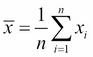where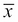is pronounced x-bar, the mathematical symbol often used to denote the mean.

To programmers coming to data science from fields outside mathematics or the sciences, this notation can be quite confusing and alienating. Others may be entirely comfortable with this notation, and they can safely skip the next section.

### Interpreting mathematical notation

Although mathematical notation may appear obscure and upsetting, there are really only a handful of symbols that will occur frequently in the formulae in this book.

Σ is pronounced sigma and means sum. When you see it in mathematical notation it means that a sequence is being added up. The symbols above and below the sigma indicate the range over which we'll be summing. They're rather like a C-style `for` loop and in the earlier formula indicate we'll be summing from i=1 up to i=n. By convention n is the length of the sequence, and sequences in mathematical notation are one-indexed, not zero-indexed, so summing from 1 to n means that we're summing over the entire length of the sequence.

The expression immediately following the sigma is the sequence to be summed. In our preceding formula for the mean, xi immediately follows the sigma. Since i will represent each index from 1 up to n, xi represents each element in the sequence of xs.

Finally,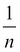appears just before the sigma, indicating that the entire expression should be multiplied by 1 divided by n (also called the reciprocal of n). This can be simplified to just dividing by n.

Name

Mathematical symbol

Clojure equivalent

n

`(count xs)`

Sigma notation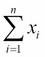`(reduce + xs)`

Pi notation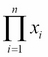`(reduce * xs)`

Putting this all together, we get "add up the elements in the sequence from the first to the last and divide by the count". In Clojure, this can be written as:

```(defn mean [xs]
(/ (reduce + xs)
(count xs)))```

Where `xs` stands for "the sequence of xs". We can use our new `mean` function to calculate the mean of the UK electorate:

```(defn ex-1-7 []
(i/\$ "Electorate")
(mean)))

;; 70149.94```

In fact, Incanter already includes a function, `mean`, to calculate the mean of a sequence very efficiently in the `incanter.stats` namespace. In this chapter (and throughout the book), the `incanter.stats` namespace will be required as `s` wherever it's used.

### The median

The median is another common descriptive statistic for measuring the central tendency of a sequence. If you ordered all the data from lowest to highest, the median is the middle value. If there is an even number of data points in the sequence, the median is usually defined as the mean of the middle two values.

The median is often represented in formulae by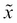, pronounced x-tilde. It's one of the deficiencies of mathematical notation that there's no particularly standard way of expressing the formula for the median value, but nonetheless it's fairly straightforward in Clojure:

```(defn median [xs]
(let [n   (count xs)
mid (int (/ n 2))]
(if (odd? n)
(nth (sort xs) mid)
(->> (sort xs)
(drop (dec mid))
(take 2)
(mean)))))```

The median of the UK electorate is:

```(defn ex-1-8 []
(i/\$ "Electorate")
(median)))

;; 70813.5```

Incanter also has a function for calculating the median value as `s/median`.

## Variance

The mean and the median are two alternative ways of describing the middle value of a sequence, but on their own they tell you very little about the values contained within it. For example, if we know the mean of a sequence of ninety-nine values is 50, we can still say very little about what values the sequence contains.

It may contain all the integers from one to ninety-nine, or forty-nine zeros and fifty ninety-nines. Maybe it contains negative one ninety-eight times and a single five-thousand and forty-eight. Or perhaps all the values are exactly fifty.

The variance of a sequence is its "spread" about the mean, and each of the preceding examples would have a different variance. In mathematical notation, the variance is expressed as: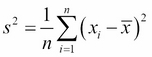where s2 is the mathematical symbol often used to denote the variance.

This equation bears a number of similarities to the equation for the mean calculated previously. Instead of summing a single value, xi, we are summing a function of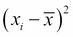. Recall that the symbolrepresents the mean value, so the function calculates the squared deviation of xi from the mean of all the xs.

We can turn the expressioninto a function, `square-deviation`, that we map over the sequence of `xs`. We can also make use of the `mean` function we've already created to sum the values in the sequence and divide by the count.

```(defn variance [xs]
(let [x-bar (mean xs)
n     (count xs)
square-deviation (fn [x]
(i/sq (- x x-bar)))]
(mean (map square-deviation xs))))```

We're using Incanter's `i/sq` function to calculate the square of our expression.

Since we've squared the deviation before taking the mean, the units of variance are also squared, so the units of the variance of the UK electorate are "people squared". This is somewhat unnatural to reason about. We can make the units more natural by taking the square root of the variance so the units are "people" again, and the result is called the standard deviation:

```(defn standard-deviation [xs]
(i/sqrt (variance xs)))

(defn ex-1-9 []
(i/\$ "Electorate")
(standard-deviation)))

;; 7672.77```

Incanter's implements functions to calculate the variance and standard deviation as `s/variance` and `s/sd` respectively.

## Quantiles

The median is one way to calculate the middle value from a list, and the variance provides a way to measure the spread of the data about this midpoint. If the entire spread of data were represented on a scale of zero to one, the median would be the value at 0.5.

For example, consider the following sequence of numbers:

`[10 11 15 21 22.5 28 30]`

There are seven numbers in the sequence, so the median is the fourth, or 21. This is also referred to as the 0.5 quantile. We can get a richer picture of a sequence of numbers by looking at the 0, 0.25, 0.5, 0.7, and 1.0 quantiles. Taken together, these numbers will not only show the median, but will also summarize the range of the data and how the numbers are distributed within it. They're sometimes referred to as the five-number summary.

One way to calculate the five-number summary for the UK electorate data is shown as follows:

```(defn quantile [q xs]
(let [n (dec (count xs))
i (-> (* n q)
(+ 1/2)
(int))]
(nth (sort xs) i)))

(defn ex-1-10 []
(i/\$ "Electorate"))
f (fn [q]
(quantile q xs))]
(map f [0 1/4 1/2 3/4 1])))

;; (21780.0 66219.0 70991.0 75115.0 109922.0)```

Quantiles can also be calculated in Incanter directly with the `s/quantile` function. A sequence of desired quantiles is passed as the keyword argument `:probs`.

### Note

Incanter's `quantile` function uses a variant of the algorithm shown earlier called the phi-quantile, which performs linear interpolation between consecutive numbers in certain cases. There are many alternative ways of calculating quantiles—consult https://en.wikipedia.org/wiki/Quantile for a discussion of the differences.

Where quantiles split the range into four equal ranges as earlier, they are called quartiles. The difference between the lower and upper quartile is referred to as the interquartile range, also often abbreviated to just IQR. Like the variance about the mean, the IQR gives a measure of the spread of the data about the median.

## Binning data

To develop an intuition for what these various calculations of variance are measuring, we can employ a technique called binning. Where data is continuous, using `frequencies` (as we did with the election data to count the nils) is not practical since no two values may be the same. However, it's possible to get a broad sense of the structure of the data by grouping the data into discrete intervals.

The process of binning is to divide the range of values into a number of consecutive, equally-sized, smaller bins. Each value in the original series falls into exactly one bin. By counting the number of points falling into each bin, we can get a sense of the spread of the data: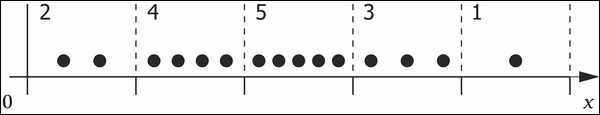The preceding illustration shows fifteen values of x split into five equally-sized bins. By counting the number of points falling into each bin we can clearly see that most points fall in the middle bin, with fewer points falling into the bins towards the edges. We can achieve the same in Clojure with the following `bin` function:

```(defn bin [n-bins xs]
(let [min-x    (apply min xs)
max-x    (apply max xs)
range-x  (- max-x min-x)
bin-fn   (fn [x]
(-> x
(- min-x)
(/ range-x)
(* n-bins)
(int)
(min (dec n-bins))))]
(map bin-fn xs)))```

For example, we can bin range 0-14 into `5` bins like so:

```(bin 5 (range 15))

;; (0 0 0 1 1 1 2 2 2 3 3 3 4 4 4)```

Once we've binned the values we can then use the `frequencies` function once again to count the number of points in each bin. In the following code, we use the function to split the UK electorate data into five bins:

```(defn ex-1-11 []
(i/\$ "Electorate")
(bin 10)
(frequencies)))

;; {1 26, 2 450, 3 171, 4 1, 0 2}```

The count of points in the extremal bins (0 and 4) is much lower than the bins in the middle—the counts seem to rise up towards the median and then down again. In the next section, we'll visualize the shape of these counts.

## Histograms

A histogram is one way to visualize the distribution of a single sequence of values. Histograms simply take a continuous distribution, bin it, and plot the frequencies of points falling into each bin as a bar. The height of each bar in the histogram represents how many points in the data are contained in that bin.

We've already seen how to bin data ourselves, but `incanter.charts` contains a `histogram` function that will bin the data and visualize it as a histogram in two steps. We require `incanter.charts` as `c` in this chapter (and throughout the book).

```(defn ex-1-12 []
(i/\$ "Electorate")
(c/histogram)
(i/view)))```

The preceding code generates the following chart: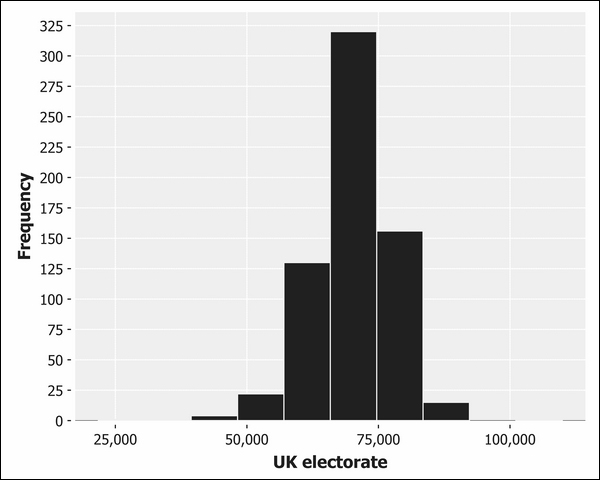We can configure the number of bins data is segmented into by passing the keyword argument `:nbins` as the second parameter to the histogram function:

```(defn ex-1-13 []
(-> (uk-electorate)
(c/histogram :nbins 200)
(i/view)))```

The preceding graph shows a single, high peak but expresses the shape of the data quite crudely. The following graph shows fine detail, but the volume of the bars obscures the shape of the distribution, particularly in the tails: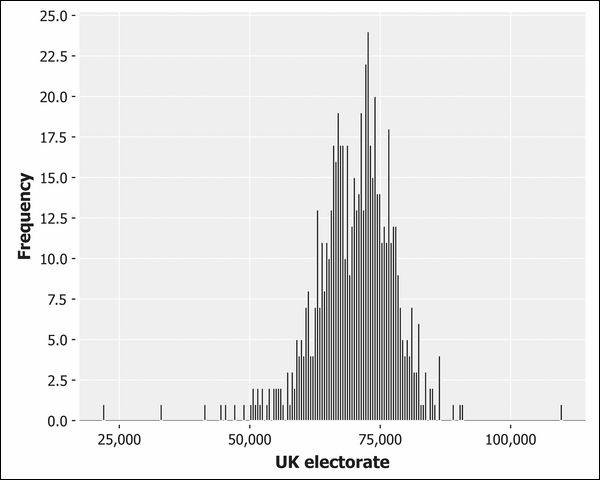Choosing the number of bins to represent your data is a fine balance—too few bins and the shape of the data will only be crudely represented, too many and noisy features may obscure the underlying structure.

```(defn ex-1-14 []
(c/histogram :x-label "UK electorate"
:nbins 20)
(i/view)))```

The following shows a histogram of `20` bars instead: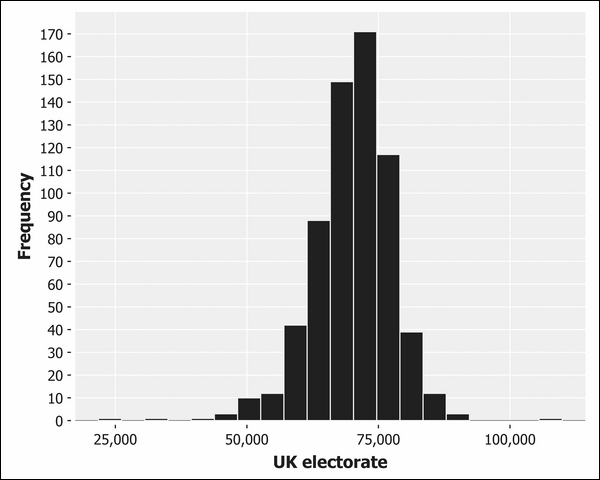This final chart containing `20` bins seems to be the best representation for this data so far.

Along with the mean and the median, the mode is another way of measuring the average value of a sequence—it's defined as the most frequently occurring value in the sequence. The mode is strictly only defined for sequences with at least one duplicated value; for many distributions, this is not the case and the mode is undefined. Nonetheless, the peak of the histogram is often referred to as the mode, since it corresponds to the most popular bin.

We can clearly see that the distribution is quite symmetrical about the mode, with values falling sharply either side along shallow tails. This is data following an approximately normal distribution.

## The normal distribution

A histogram will tell you approximately how data is distributed throughout its range, and provide a visual means of classifying your data into one of a handful of common distributions. Many distributions occur frequently in data analysis, but none so much as the normal distribution, also called the Gaussian distribution.

### Note

The distribution is named the normal distribution because of how often it occurs in nature. Galileo noticed that the errors in his astronomical measurements followed a distribution where small deviations from the mean occurred more frequently than large deviations. It was the great mathematician Gauss' contribution to describing the mathematical shape of these errors that led to the distribution also being called the Gaussian distribution in his honor.

A distribution is like a compression algorithm: it allows a potentially large amount of data to be summarized very efficiently. The normal distribution requires just two parameters from which the rest of the data can be approximated—the mean and the standard deviation.

### The central limit theorem

The reason for the normal distribution's ubiquity is partly explained by the central limit theorem. Values generated from diverse distributions will tend to converge to the normal distribution under certain circumstances, as we will show next.

A common distribution in programming is the uniform distribution. This is the distribution of numbers generated by Clojure's `rand` function: for a fair random number generator, all numbers have an equal chance of being generated. We can visualize this on a histogram by generating a random number between zero and one many times over and plotting the results.

```(defn ex-1-15 []
(let [xs (->> (repeatedly rand)
(take 10000))]
(-> (c/histogram xs
:x-label "Uniform distribution"
:nbins 20)
(i/view))))```

The preceding code will generate the following histogram: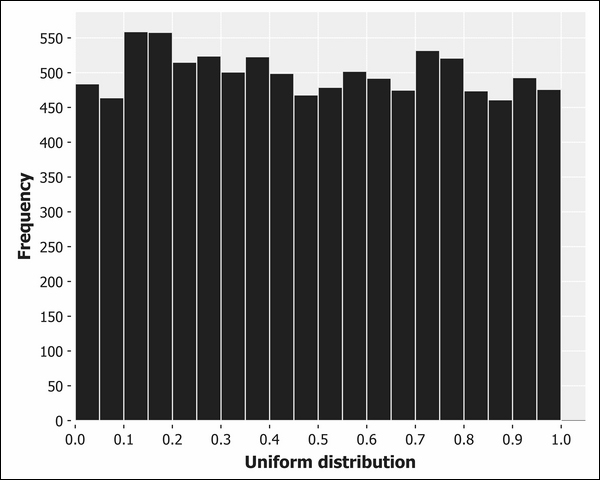Each bar of the histogram is approximately the same height, corresponding to the equal probability of generating a number that falls into each bin. The bars aren't exactly the same height since the uniform distribution describes the theoretical output that our random sampling can't mirror precisely. Over the next several chapters, we'll learn ways to precisely quantify the difference between theory and practice to determine whether the differences are large enough to be concerned with. In this case, they are not.

If instead we generate a histogram of the means of sequences of numbers, we'll end up with a distribution that looks rather different.

```(defn ex-1-16 []
(let [xs (->> (repeatedly rand)
(partition 10)
(map mean)
(take 10000))]
(-> (c/histogram xs
:x-label "Distribution of means"
:nbins 20)
(i/view))))```

The preceding code will provide an output similar to the following histogram: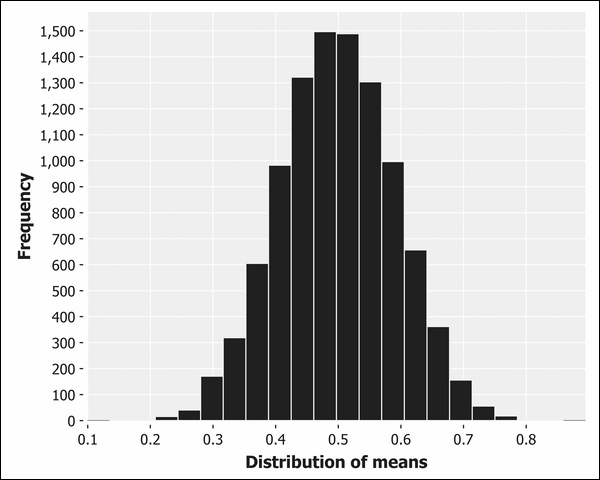Although it's not impossible for the mean to be close to zero or one, it's exceedingly improbable and grows less probable as both the number of averaged numbers and the number of sampled averages grow. In fact, the output is exceedingly close to the normal distribution.

This outcome—where the average effect of many small random fluctuations leads to the normal distribution—is called the central limit theorem, sometimes abbreviated to CLT, and goes a long way towards explaining why the normal distribution occurs so frequently in natural phenomena.

The central limit theorem wasn't named until the 20th century, although the effect had been documented as early as 1733 by the French mathematician Abraham de Moivre, who used the normal distribution to approximate the number of heads resulting from tosses of a fair coin. The outcome of coin tosses is best modeled with the binomial distribution, which we will introduce in Chapter 4, Classification. While the central limit theorem provides a way to generate samples from an approximate normal distribution, Incanter's `distributions` namespace provides functions for generating samples efficiently from a variety of distributions, including the normal:

```(defn ex-1-17 []
(let [distribution (d/normal-distribution)
xs (->> (repeatedly #(d/draw distribution))
(take 10000))]
(-> (c/histogram xs
:x-label "Normal distribution"
:nbins 20)
(i/view))))```

The preceding code generates the following histogram: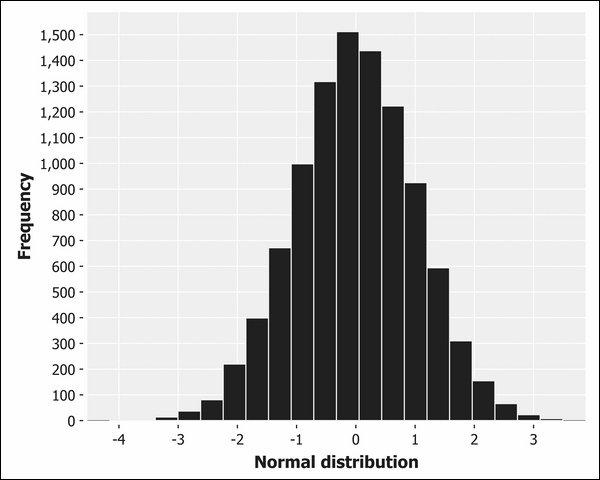The `d/draw` function will return one sample from the supplied distribution. The default mean and standard deviation from `d/normal-distribution` are zero and one respectively.

## Poincaré's baker

There's a story that, while almost certainly apocryphal, allows us to look in more detail at the way in which the central limit theorem allows us to reason about how distributions are formed. It concerns the celebrated nineteenth century French polymath Henri Poincaré who, so the story goes, weighed his bread every day for a year.

Baking was a regulated profession, and Poincaré discovered that, while the weights of the bread followed a normal distribution, the peak was at 950g rather than the advertised 1kg. He reported his baker to the authorities and so the baker was fined.

The next year, Poincaré continued to weigh his bread from the same baker. He found the mean value was now 1kg, but that the distribution was no longer symmetrical around the mean. The distribution was skewed to the right, consistent with the baker giving Poincaré only the heaviest of his loaves. Poincaré reported his baker to the authorities once more and his baker was fined a second time.

Whether the story is true or not needn't concern us here; it's provided simply to illustrate a key point—the distribution of a sequence of numbers can tell us something important about the process that generated it.

### Generating distributions

To develop our intuition about the normal distribution and variance, let's model an honest and dishonest baker using Incanter's distribution functions. We can model the honest baker as a normal distribution with a mean of 1,000, corresponding to a fair loaf of 1kg. We'll assume a variance in the baking process that results in a standard deviation of 30g.

```(defn honest-baker [mean sd]
(let [distribution (d/normal-distribution mean sd)]
(repeatedly #(d/draw distribution))))

(defn ex-1-18 []
(-> (take 10000 (honest-baker 1000 30))
(c/histogram :x-label "Honest baker"
:nbins 25)
(i/view)))```

The preceding code will provide an output similar to the following histogram: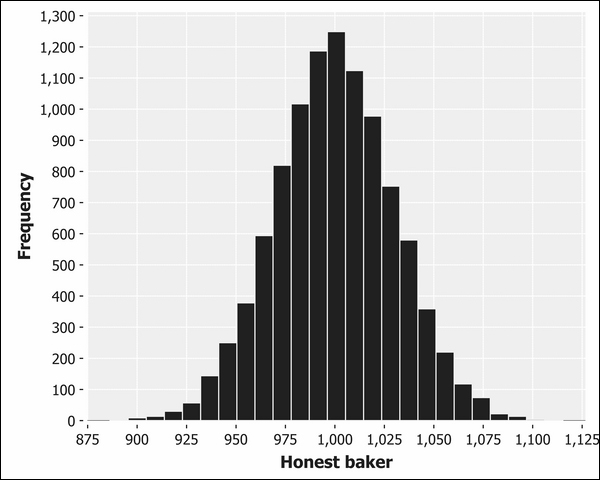Now, let's model a baker who sells only the heaviest of his loaves. We partition the sequence into groups of thirteen (a "baker's dozen") and pick the maximum value:

```(defn dishonest-baker [mean sd]
(let [distribution (d/normal-distribution mean sd)]
(->> (repeatedly #(d/draw distribution))
(partition 13)
(map (partial apply max)))))

(defn ex-1-19 []
(-> (take 10000 (dishonest-baker 950 30))
(c/histogram :x-label "Dishonest baker"
:nbins 25)
(i/view)))```

The preceding code will produce a histogram similar to the following: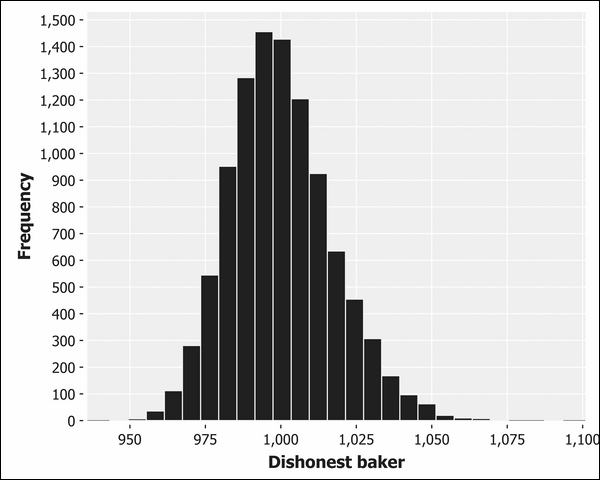It should be apparent that this histogram does not look quite like the others we have seen. The mean value is still 1kg, but the spread of values around the mean is no longer symmetrical. We say that this histogram indicates a skewed normal distribution.

## Skewness

Skewness is the name for the asymmetry of a distribution about its mode. Negative skew, or left skew, indicates that the area under the graph is larger on the left side of the mode. Positive skew, or right skew, indicates that the area under the graph is larger on the right side of the mode.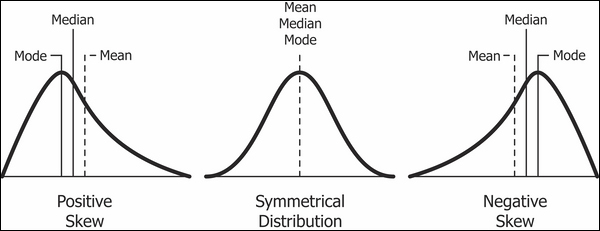Incanter has a built-in function for measuring skewness in the `stats` namespace:

```(defn ex-1-20 []
(let [weights (take 10000 (dishonest-baker 950 30))]
{:mean (mean weights)
:median (median weights)
:skewness (s/skewness weights)}))```

The preceding example shows that the skewness of the dishonest baker's output is about 0.4, quantifying the skew evident in the histogram.

### Quantile-quantile plots

We encountered quantiles as a means of describing the distribution of data earlier in the chapter. Recall that the `quantile` function accepts a number between zero and one and returns the value of the sequence at that point. 0.5 corresponds to the median value.

Plotting the quantiles of your data against the quantiles of the normal distribution allows us to see how our measured data compares against the theoretical distribution. Plots such as this are called Q-Q plots and they provide a quick and intuitive way of determining normality. For data corresponding closely to the normal distribution, the Q-Q Plot is a straight line. Deviations from a straight line indicate the manner in which the data deviates from the idealized normal distribution.

Let's plot Q-Q plots for both our honest and dishonest bakers side-by-side. Incanter's `c/qq-plot` function accepts the list of data points and generates a scatter chart of the sample quantiles plotted against the quantiles from the theoretical normal distribution:

```(defn ex-1-21 []
(->> (honest-baker 1000 30)
(take 10000)
(c/qq-plot)
(i/view))
(->> (dishonest-baker 950 30)
(take 10000)
(c/qq-plot)
(i/view)))```

The preceding code will produce the following plots: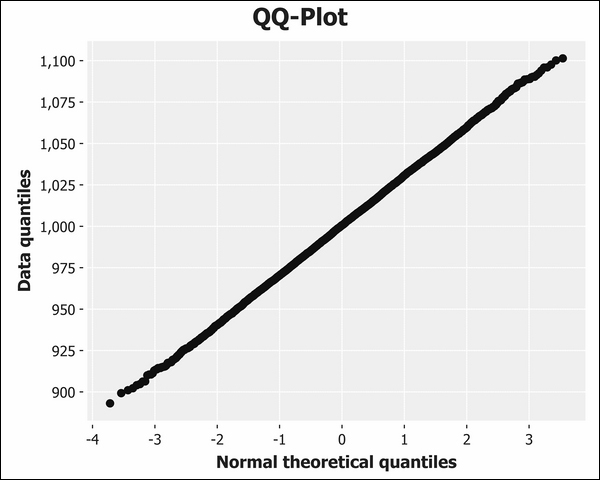The Q-Q plot for the honest baker is shown earlier. The dishonest baker's plot is next: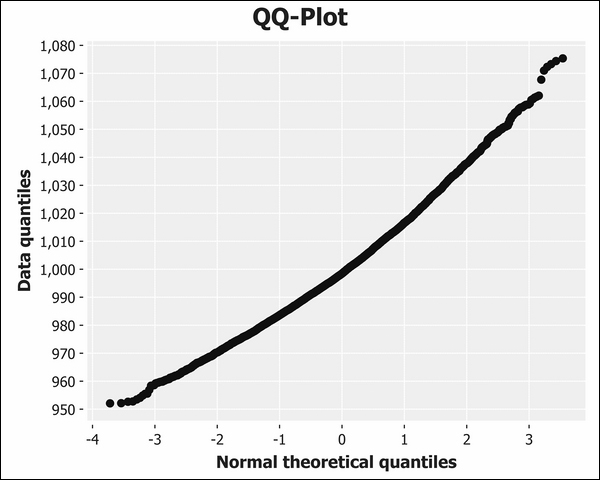The fact that the line is curved indicates that the data is positively skewed; a curve in the other direction would indicate negative skew. In fact, Q-Q plots make it easier to discern a wide variety of deviations from the standard normal distribution, as shown in the following diagram: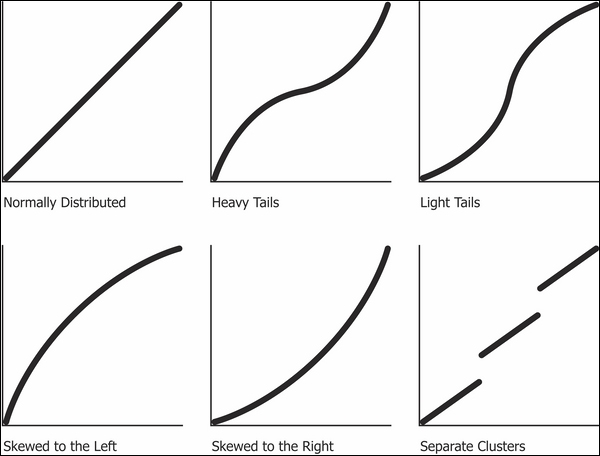Q-Q plots compare the distribution of the honest and dishonest baker against the theoretical normal distribution. In the next section, we'll compare several alternative ways of visually comparing two (or more) measured sequences of values with each other.

## Comparative visualizations

Q-Q plots provide a great way to compare a measured, empirical distribution to a theoretical normal distribution. If we'd like to compare two or more empirical distributions with each other, we can't use Incanter's Q-Q plot charts. We have a variety of other options, though, as shown in the next two sections.

### Box plots

Box plots, or box and whisker plots, are a way to visualize the descriptive statistics of median and variance visually. We can generate them using the following code:

```(defn ex-1-22 []
(-> (c/box-plot (->> (honest-baker 1000 30)
(take 10000))
:legend true
:y-label "Loaf weight (g)"
:series-label "Honest baker")
(take 10000))
:series-label "Dishonest baker")
(i/view)))```

This creates the following plot: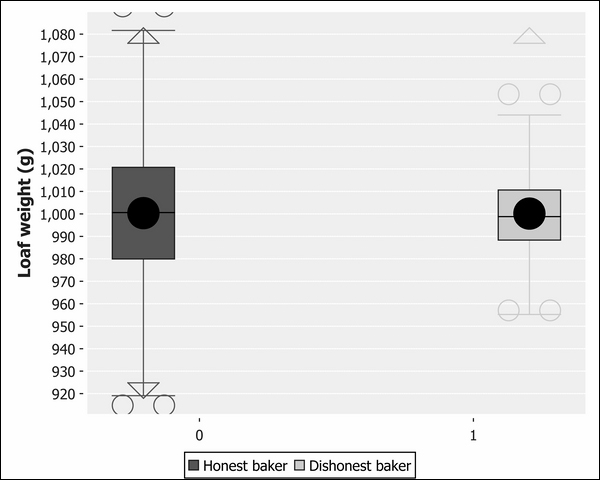The boxes in the center of the plot represent the interquartile range. The median is the line across the middle of the box, and the mean is the large black dot. For the honest baker, the median passes through the centre of the circle, indicating the mean and median are about the same. For the dishonest baker, the mean is offset from the median, indicating a skew.

The whiskers indicate the range of the data and outliers are represented by hollow circles. In just one chart, we're more clearly able to see the difference between the two distributions than we were on either the histograms or the Q-Q plots independently.

### Cumulative distribution functions

Cumulative distribution functions, also known as CDFs, describe the probability that a value drawn from a distribution will have a value less than x. Like all probability distributions, they value between 0 and 1, with 0 representing impossibility and 1 representing certainty. For example, imagine that I'm about to throw a six-sided die. What's the probability that I'll roll less than a six?

For a fair die, the probability I'll row a five or lower is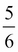. Conversely, the probability I'll roll a one is only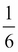. Three or lower corresponds to even odds—a probability of 50 percent.

The CDF of die rolls follows the same pattern as all CDFs—for numbers at the lower end of the range, the CDF is close to zero, corresponding to a low probability of selecting numbers in this range or below. At the high end of the range, the CDF is close to one, since most values drawn from the sequence will be lower.

### Note

The CDF and quantiles are closely related to each other—the CDF is the inverse of the quantile function. If the 0.5 quantile corresponds to a value of 1,000, then the CDF for 1,000 is 0.5.

Just as Incanter's `s/quantile` function allows us to sample values from a distribution at specific points, the `s/cdf-empirical` function allows us to input a value from the sequence and return a value between zero and one. It is a higher-order function—one that will accept the value (in this case, a sequence of values) and return a function. The returned function can then be called as often as necessary with different input values, returning the CDF for each of them.

### Note

Higher-order functions are functions that accept or return functions.

Let's plot the CDF of both the honest and dishonest bakers side by side. We can use Incanter's `c/xy-plot` for visualizing the CDF by plotting the source data—the samples from our honest and dishonest bakers—against the probabilities calculated against the empirical CDF. The `c/xy-plot` function expects the x values and the y values to be supplied as two separate sequences of values.

To plot both distributions on the same chart, we need to be able to provide multiple series to our `xy-plot`. Incanter offers functions for many of its charts to add additional series. In the case of an `xy-plot`, we can use the function `c/add-lines`, which accepts the chart as the first argument, and the x series and the y series of data as the next two arguments respectively. You can also pass an optional series label. We do this in the following code so we can tell the two series apart on the finished chart:

```(defn ex-1-23 []
(let [sample-honest    (->> (honest-baker 1000 30)
(take 1000))
sample-dishonest (->> (dishonest-baker 950 30)
(take 1000))
ecdf-honest    (s/cdf-empirical sample-honest)
ecdf-dishonest (s/cdf-empirical sample-dishonest)]
(-> (c/xy-plot sample-honest (map ecdf-honest sample-honest)
:x-label "Loaf Weight"
:y-label "Probability"
:legend true
:series-label "Honest baker")
(map ecdf-dishonest sample-dishonest)
:series-label "Dishonest baker")
(i/view))))```

The preceding code generates the following chart: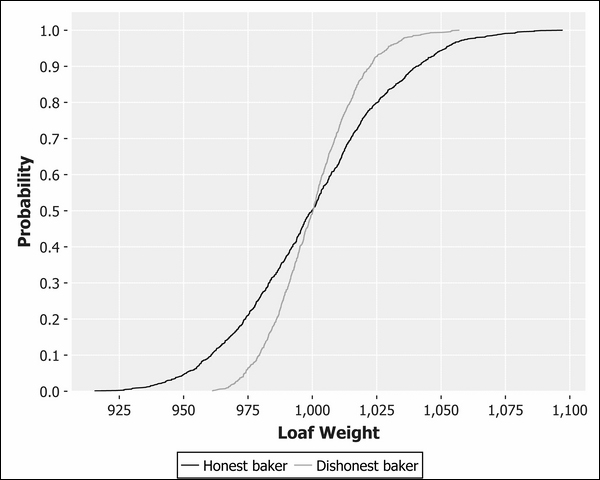Although it looks very different, this chart shows essentially the same information as the box and whisker plot. We can see that the two lines cross at approximately the median of 0.5, corresponding to 1,000g. The dishonest line is truncated at the lower tail and longer on the upper tail, corresponding to a skewed distribution.

## The importance of visualizations

Simple visualizations like those earlier are succinct ways of conveying a large quantity of information. They complement the summary statistics we calculated earlier in the chapter, and it's important that we use them. Statistics such as the mean and standard deviation necessarily conceal a lot of information as they reduce a sequence down to just a single number.

The statistician Francis Anscombe devised a collection of four scatter plots, known as Anscombe's Quartet, that have nearly identical statistical properties (including the mean, variance, and standard deviation). In spite of this, it's visually apparent that the distribution of xs and ys are all very different: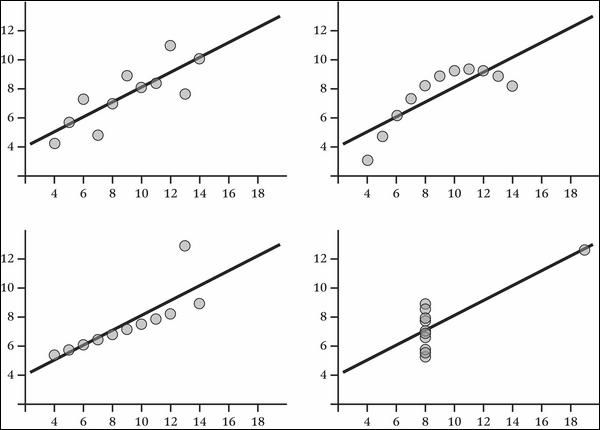Datasets don't have to be contrived to reveal valuable insights when graphed. Take for example this histogram of the marks earned by candidates in Poland's national Matura exam in 2013: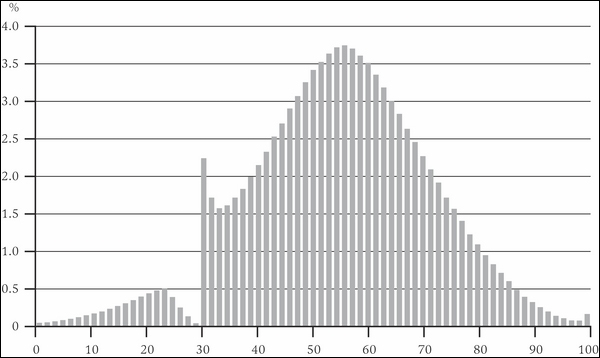We might expect the abilities of students to be normally distributed and indeed—with the exception of a sharp spike around 30 percent —it is. What we can clearly see is the very human effect of examiners nudging student's grades over the pass mark.

In fact, the distributions for sequences drawn from large samples can be so reliable that any deviation from them can be evidence of illegal activity. Benford's law, also called the first-digit law, is a curious feature of random numbers generated over a large range. One occurs as the leading digit about 30 percent of the time, while larger digits occur less and less frequently. For example, nine occurs as the leading digit less than 5 percent of the time.

### Note

Benford's law is named after physicist Frank Benford who stated it in 1938 and showed its consistency across a wide variety of data sources. It had been previously observed by Simon Newcomb over 50 years earlier, who noticed that the pages of his books of logarithm tables were more battered for numbers beginning with the digit one.

Benford showed that the law applied to data as diverse as electricity bills, street addresses, stock prices, population numbers, death rates, and lengths of rivers. The law is so consistent for data sets covering large ranges of values that deviation from it has been accepted as evidence in trials for financial fraud.

### Visualizing electorate data

Let's return to the election data and compare the electorate sequence we created earlier against the theoretical normal distribution CDF. We can use Incanter's `s/cdf-normal` function to generate a normal CDF from a sequence of values. The default mean is 0 and standard deviation is 1, so we'll need to provide the measured mean and standard deviation from the electorate data. These values for our electorate data are 70,150 and 7,679, respectively.

We generated an empirical CDF earlier in the chapter. The following example simply generates each of the two CDFs and plots them on a single `c/xy-plot`:

```(defn ex-1-24 []
(i/\$ "Electorate"))
ecdf   (s/cdf-empirical electorate)
fitted (s/cdf-normal electorate
:mean (s/mean electorate)
:sd   (s/sd electorate))]
(-> (c/xy-plot electorate fitted
:x-label "Electorate"
:y-label "Probability"
:series-label "Fitted"
:legend true)
:series-label "Empirical")
(i/view))))```

The preceding example generates the following plot: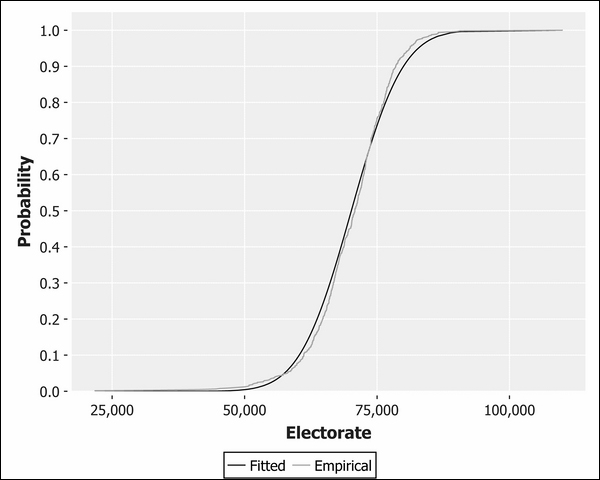You can see from the proximity of the two lines to each other how closely this data resembles normality, although a slight skew is evident. The skew is in the opposite direction to the dishonest baker CDF we plotted previously, so our electorate data is slightly skewed to the left.

As we're comparing our distribution against the theoretical normal distribution, let's use a Q-Q plot, which will do this by default:

```(defn ex-1-25 []
(i/\$ "Electorate")
(c/qq-plot)
(i/view)))```

The following Q-Q plot does an even better job of highlighting the left skew evident in the data: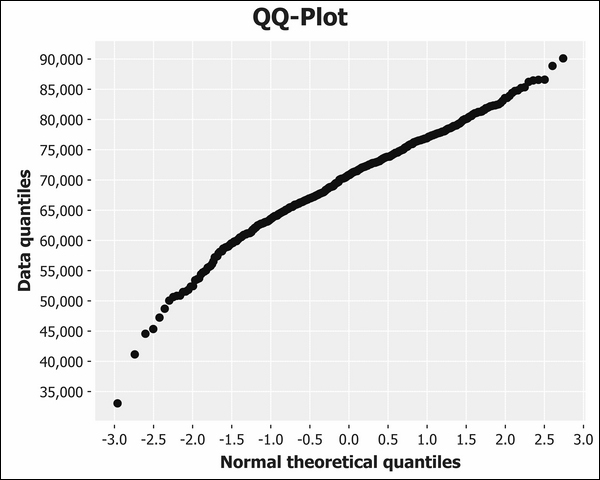As we expected, the curve bows in the opposite direction to the dishonest baker Q-Q plot earlier in the chapter. This indicates that there is a greater number of constituencies that are smaller than we would expect if the data were more closely normally distributed.

So far this chapter, we've reduced the size of our dataset by filtering both rows and columns. Often we'll want to add rows to a dataset instead, and Incanter supports this in several ways.

Firstly, we can choose whether to replace an existing column within the dataset or append an additional column to the dataset. Secondly, we can choose whether to supply the new column values to replace the existing column values directly, or whether to calculate the new values by applying a function to each row of the data.

The following chart lists our options and the corresponding Incanter function to use:

Replace data

Append data

By providing a sequence

`i/replace-column`

`i/add-column`

By applying a function

`i/transform-column`

`i/add-derived-column`

When transforming or deriving a column based on a function, we pass the name of the new column to create, a function to apply for each row, and also a sequence of existing column names. The values contained in each of these existing columns will comprise the arguments to our function.

Let's show how to use the `i/add-derived-column` function with reference to a real example. The 2010 UK general election resulted in a hung parliament with no single party commanding an overall majority. A coalition between the Conservative and Liberal Democrat parties was formed. In the next section we'll find out how many people voted for either party, and what percentage of the total vote this was.

To find out what percentage of the electorate voted for either the Conservative or Liberal Democrat parties, we'll want to calculate the sum of votes for either party. Since we're creating a new field of data based on a function of the existing data, we'll want to use the `i/add-derived-column` function.

```(defn ex-1-26 []

If we run this now, however, an exception will be generated:

`ClassCastException java.lang.String cannot be cast to java.lang.Number  clojure.lang.Numbers.add (Numbers.java:126)`

Unfortunately Clojure is complaining that we're trying to add a `java.lang.String`. Clearly either (or both) the `Con` or the `LD` columns contain string values, but which? We can use frequencies again to see the extent of the problem:

```(->> (load-data :uk-scrubbed)
(\$ "Con")
(map type)
(frequencies))

;; {java.lang.Double 631, java.lang.String 19}

(\$ "LD")
(map type)
(frequencies))

;; {java.lang.Double 631, java.lang.String 19}```

Let's use the `i/\$where` function we encountered earlier in the chapter to inspect just these rows:

```(defn ex-1-27 []
(i/\$where #(not-any? number? [(% "Con") (% "LD")]))
(i/\$ [:Region :Electorate :Con :LD])))

;; |           Region | Electorate | Con | LD |
;; |------------------+------------+-----+----|
;; | Northern Ireland |    60204.0 |     |    |
;; | Northern Ireland |    73338.0 |     |    |
;; | Northern Ireland |    63054.0 |     |    |
;; ...```

This bit of exploration should be enough to convince us that the reason for these fields being blank is that candidates were not put forward in the corresponding constituencies. Should they be filtered out or assumed to be zero? This is an interesting question. Let's filter them out, since it wasn't even possible for voters to choose a Liberal Democrat or Conservative candidate in these constituencies. If instead we assumed a zero, we would artificially lower the mean number of people who—given the choice—voted for either of these parties.

Now that we know how to filter the problematic rows, let's add the derived columns for the victor and the victor's share of the vote, along with election turnout. We filter the rows to show only those where both a Conservative and Liberal Democrat candidate were put forward:

```(defmethod load-data :uk-victors [_]
(i/\$where {:Con {:\$fn number?} :LD {:\$fn number?}})

As a result, we now have three additional columns in our dataset: `:victors`, `:victors-share`, and `:turnout`. Let's plot the victor's share of the vote as a Q-Q plot to see how it compares against the theoretical normal distribution:

```(defn ex-1-28 []
(i/\$ :victors-share)
(c/qq-plot)
(i/view)))```

The preceding code generates the following plot: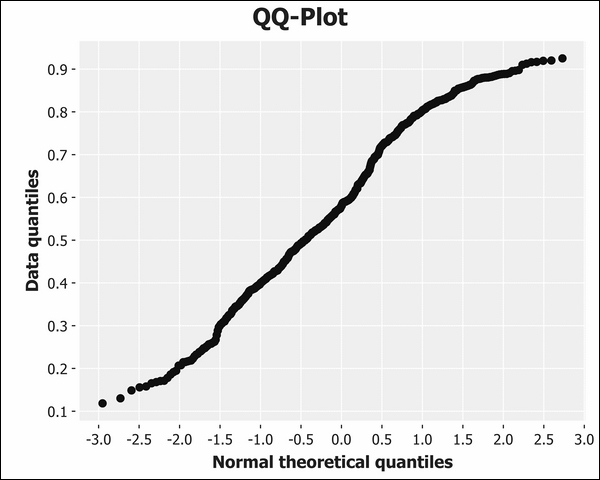Referring back to the diagram of various Q-Q plot shapes earlier in the chapter reveals that the victor's share of the vote has "light tails" compared to the normal distribution. This means that more of the data is closer to the mean than we might expect from truly normally distributed data.

## Comparative visualizations of electorate data

Let's look now at a dataset from another general election, this time from Russia in 2011. Russia is a much larger country, and its election data is much larger too. We'll be loading two large Excel files into the memory, which may exceed your default JVM heap size.

To expand the amount of memory available to Incanter, we can adjust the JVM settings in the project's `profile.clj`. The a vector of configuration flags for the JVM can be provided with the key `:jvm-opts`. Here we're using Java's `Xmx` flag to increase the heap size to 1GB. This should be more than enough.

`  :jvm-opts ["-Xmx1G"]`

Russia's data is available in two data files. Fortunately the columns are the same in each, so they can be concatenated together end-to-end. Incanter's function `i/conj-rows` exists for precisely this purpose:

```(defmethod load-data :ru [_]
(i/conj-rows (-> (io/resource "Russia2011_1of2.xls")
(str)
(-> (io/resource "Russia2011_2of2.xls")
(str)

In the preceding code, we define a third implementation of the `load-data` multimethod to load and combine both Russia files.

### Note

In addition to `conj-rows`, Incanter-core also defines `conj-columns` that will merge the columns of datasets provided they have the same number of rows.

Let's see what the Russia data column names are:

```(defn ex-1-29 []
(i/col-names)))

;; ["Code for district"
;; "Number of the polling district (unique to state, not overall)"
;; "Name of district" "Number of voters included in voters list"
;; "The number of ballots received by the precinct election
;; commission" ...]```

The column names in the Russia dataset are very descriptive, but perhaps longer than we want to type out. Also, it would be convenient if columns that represent the same attributes as we've already seen in the UK election data (the victor's share and turnout for example) were labeled the same in both datasets. Let's rename them accordingly.

Along with a dataset, the `i/rename-cols` function expects to receive a map whose keys are the current column names with values corresponding to the desired new column name. If we combine this with the `i/add-derived-column` data we have already seen, we arrive at the following:

```(defmethod load-data :ru-victors [_]
(i/rename-cols
{"Number of voters included in voters list" :electorate
"Number of valid ballots" :valid-ballots
"United Russia" :victors})
[:victors :valid-ballots] i/safe-div)
[:valid-ballots :electorate] /)))```

The `i/safe-div` function is identical to `/` but will protect against division by zero. Rather than raising an exception, it returns the value `Infinity`, which will be ignored by Incanter's statistical and charting functions.

## Visualizing the Russian election data

We previously saw that a histogram of the UK election turnout was approximately normal (albeit with light tails). Now that we've loaded and transformed the Russian election data, let's see how it compares:

```(defn ex-1-30 []
(c/histogram :x-label "Russia turnout"
:nbins 20)
(i/view)))```

The preceding example generates the following histogram: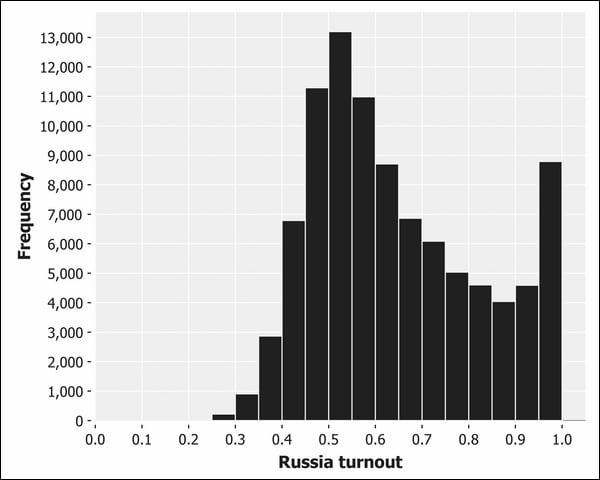This histogram doesn't look at all like the classic bell-shaped curves we've seen so far. There's a pronounced positive skew, and the voter turnout actually increases from 80 percent towards 100 percent—the opposite of what we would expect from normally-distributed data.

Given the expectations set by the UK data and by the central limit theorem, this is a curious result. Let's visualize the data with a Q-Q plot instead:

```(defn ex-1-31 []
(i/\$ :turnout)
(c/qq-plot)
(i/view)))```

This returns the following plot: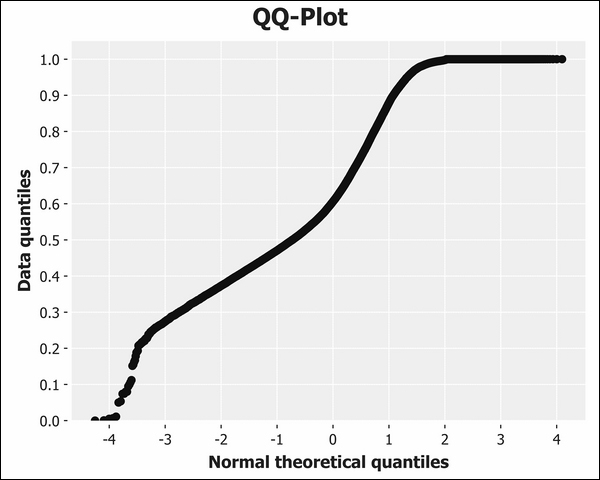This Q-Q plot is neither a straight line nor a particularly S-shaped curve. In fact, the Q-Q plot suggests a light tail at the top end of the distribution and a heavy tail at the bottom. This is almost the opposite of what we see on the histogram, which clearly indicates an extremely heavy right tail.

In fact, it's precisely because the tail is so heavy that the Q-Q plot is misleading: the density of points between 0.5 and 1.0 on the histogram suggests that the peak should be around 0.7 with a right tail continuing beyond 1.0. It's clearly illogical that we would have a percentage exceeding 100 percent but the Q-Q plot doesn't account for this (it doesn't know we're plotting percentages), so the sudden absence of data beyond 1.0 is interpreted as a clipped right tail.

Given the central limit theorem, and what we've observed with the UK election data, the tendency towards 100 percent voter turnout is curious. Let's compare the UK and Russia datasets side-by-side.

## Comparative visualizations

Let's suppose we'd like to compare the distributions of electorate data between the UK and Russia. We've already seen in this chapter how to make use of CDFs and box plots, so let's investigate an alternative that's similar to a histogram.

We could try and plot both datasets on a histogram but this would be a bad idea. We wouldn't be able to interpret the results for two reasons:

• The sizes of the voting districts, and therefore the means of the distributions, are very different

• The number of voting districts overall is so different, so the histograms bars will have different heights

An alternative to the histogram that addresses both of these issues is the probability mass function (PMF).

### Probability mass functions

The probability mass function, or PMF, has a lot in common with a histogram. Instead of plotting the counts of values falling into bins, though, it instead plots the probability that a number drawn from a distribution will be exactly equal to a given value. As the function assigns a probability to every value that can possibly be returned by the distribution, and because probabilities are measured on a scale from zero to one, (with one corresponding to certainty), the area under the probability mass function is equal to one.

Thus, the PMF ensures that the area under our plots will be comparable between datasets. However, we still have the issue that the sizes of the voting districts—and therefore the means of the distributions—can't be compared. This can be addressed by a separate technique—normalization.

### Note

Normalizing the data isn't related to the normal distribution. It's the name given to the general task of bringing one or more sequences of values into alignment. Depending on the context, it could mean simply adjusting the values so they fall within the same range, or more sophisticated procedures to ensure that the distributions of data are the same. In general, the goal of normalization is to facilitate the comparison of two or more series of data.

There are innumerable ways to normalize data, but one of the most basic is to ensure that each series is in the range zero to one. None of our values decrease below zero, so we can accomplish this normalization by simply dividing by the largest value:

```(defn as-pmf [bins]
(let [histogram (frequencies bins)
total     (reduce + (vals histogram))]
(->> histogram
(map (fn [[k v]]
[k (/ v total)]))
(into {}))))```

With the preceding function in place, we can normalize both the UK and Russia data and plot it side by side on the same axes:

```(defn ex-1-32 []
(let [n-bins 40
(i/\$ :turnout)
(bin n-bins)
(as-pmf))
(i/\$ :turnout)
(bin n-bins)
(as-pmf))]
(-> (c/xy-plot (keys uk) (vals uk)
:series-label "UK"
:legend true
:x-label "Turnout Bins"
:y-label "Probability")
:series-label "Russia")
(i/view))))```

The preceding example generates the following chart: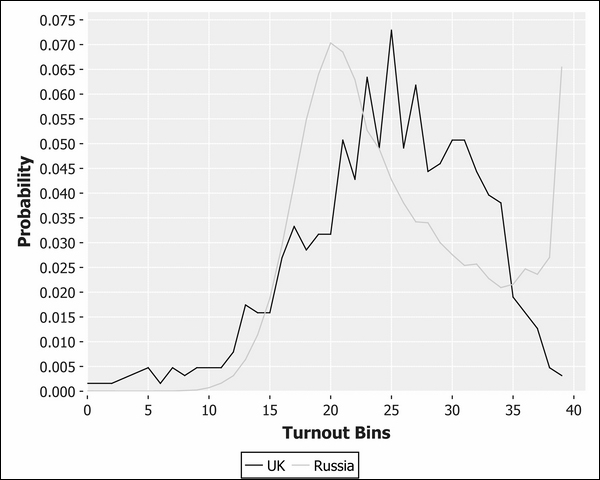After normalization, the two distributions can be compared more readily. It's clearly apparent how—in spite of having a lower mean turnout than the UK—the Russia election had a massive uplift towards 100-percent turnout. Insofar as it represents the combined effect of many independent choices, we would expect election results to conform to the central limit theorem and be approximately normally distributed. In fact, election results from around the world generally conform to this expectation.

Although not quite as high as the modal peak in the center of the distribution—corresponding to approximately 50 percent turnout—the Russian election data presents a very anomalous result. Researcher Peter Klimek and his colleagues at the Medical University of Vienna have gone as far as to suggest that this is a clear signature of ballot-rigging.

### Scatter plots

We've observed the curious results for the turnout at the Russian election and identified that it has a different signature from the UK election. Next, let's see how the proportion of votes for the winning candidate is related to the turnout. After all, if the unexpectedly high turnout really is a sign of foul play by the incumbent government, we'd anticipate that they'll be voting for themselves rather than anyone else. Thus we'd expect most, if not all, of these additional votes to be for the ultimate election winners.

Chapter 3, Correlation, will cover the statistics behind correlating two variables in much more detail, but for now it would be interesting simply to visualize the relationship between turnout and the proportion of votes for the winning party.

The final visualization we'll introduce this chapter is the scatter plot. Scatter plots are very useful for visualizing correlations between two variables: where a linear correlation exists, it will be evident as a diagonal tendency in the scatter plot. Incanter contains the `c/scatter-plot` function for this kind of chart with arguments the same as for the `c/xy-plot` function.

```(defn ex-1-33 []
(-> (c/scatter-plot (i/\$ :turnout data)
(i/\$ :victors-share data)
:x-label "Turnout"
:y-label "Victor's Share")
(i/view))))```

The preceding code generates the following chart: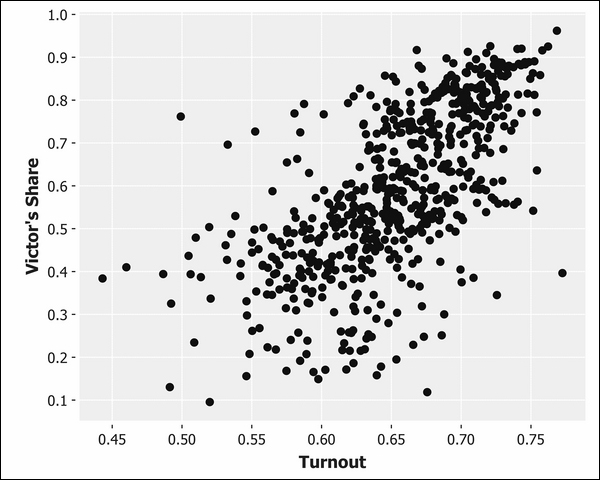Although the points are arranged broadly in a fuzzy ellipse, a diagonal tendency towards the top right of the scatter plot is clearly apparent. This indicates an interesting result—turnout is correlated with the proportion of votes for the ultimate election winners. We might have expected the reverse: voter complacency leading to a lower turnout where there was a clear victor in the running.

### Note

As mentioned earlier, the UK election of 2010 was far from ordinary, resulting in a hung parliament and a coalition government. In fact, the "winners" in this case represent two parties who had, up until election day, been opponents. A vote for either counts as a vote for the winners.

Next, we'll create the same scatter plot for the Russia election:

```(defn ex-1-34 []
(-> (c/scatter-plot (i/\$ :turnout data)
(i/\$ :victors-share data)
:x-label "Turnout"
:y-label "Victor's Share")
(i/view))))```

This generates the following plot: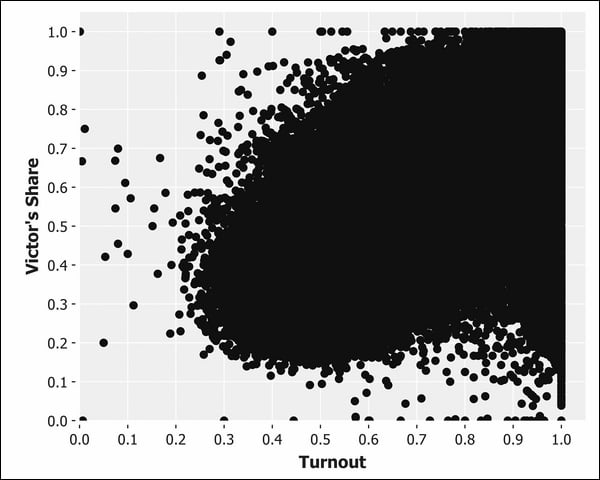Although a diagonal tendency in the Russia data is clearly evident from the outline of the points, the sheer volume of data obscures the internal structure. In the last section of this chapter, we'll show a simple technique for extracting structure from a chart such as the earlier one using opacity.

### Scatter transparency

In situations such as the preceding one where a scatter plot is overwhelmed by the volume of points, transparency can help to visualize the structure of the data. Since translucent points that overlap will be more opaque, and areas with fewer points will be more transparent, a scatter plot with semi-transparent points can show the density of the data much better than solid points can.

We can set the alpha transparency of points plotted on an Incanter chart with the `c/set-alpha` function. It accepts two arguments: the chart and a number between zero and one. One signifies fully opaque and zero fully transparent.

```(defn ex-1-35 []
(s/sample :size 10000))]
(-> (c/scatter-plot (i/\$ :turnout data)
(i/\$ :victors-share data)
:x-label "Turnout"
:y-label "Victor Share")
(c/set-alpha 0.05)
(i/view))))```

The preceding example generates the following chart: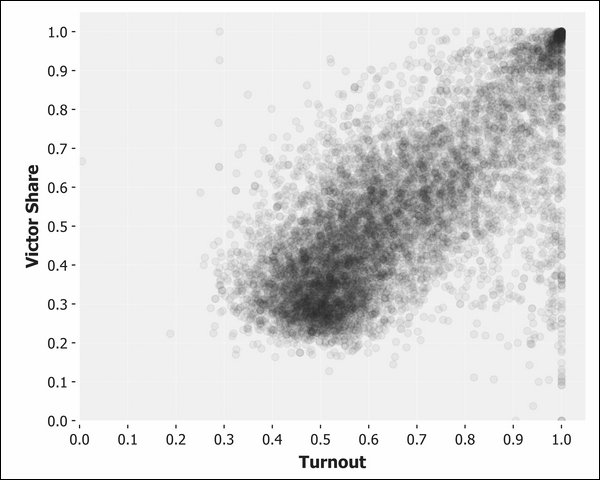The preceding scatter plot shows the general tendency of the victor's share and the turnout to vary together. We can see a correlation between the two values, and a "hot spot" in the top right corner of the chart corresponding to close to 100-percent turnout and 100-percent votes for the winning party. This in particular is the sign that the researchers at the Medial University of Vienna have highlighted as being the signature of electoral fraud. It's evident in the results of other disputed elections around the world, such as those of the 2011 Ugandan presidential election, too.

### Tip

The district-level results for many other elections around the world are available at http://www.complex-systems.meduniwien.ac.at/elections/election.html. Visit the site for links to the research paper and to download other datasets on which to practice what you've learned in this chapter about scrubbing and transforming real data.

We'll cover correlation in more detail in Chapter 3, Correlation, when we'll learn how to quantify the strength of the relationship between two values and build a predictive model based on it. We'll also revisit this data in Chapter 10, Visualization when we implement a custom two-dimensional histogram to visualize the relationship between turnout and the winner's proportion of the vote even more clearly.

## Summary

In this first chapter, we've learned about summary statistics and the value of distributions. We've seen how even a simple analysis can provide evidence of potentially fraudulent activity.

In particular, we've encountered the central limit theorem and seen why it goes such a long way towards explaining the ubiquity of the normal distribution throughout data science. An appropriate distribution can represent the essence of a large sequence of numbers in just a few statistics and we've implemented several of them using pure Clojure functions in this chapter. We've also introduced the Incanter library and used it to load, transform, and visually compare several datasets. We haven't been able to do much more than note a curious difference between two distributions, however.

In the next chapter, we'll extend what we've learned about descriptive statistics to cover inferential statistics. These will allow us to quantify a measured difference between two or more distributions and decide whether a difference is statistically significant. We'll also learn about hypothesis testing—a framework for conducting robust experiments that allow us to draw conclusions from data.

• Henry Garner

Henry Garner is a graduate from the University of Oxford and an experienced developer, CTO, and coach.

He started his technical career at Britain's largest telecoms provider, BT, working with a traditional data warehouse infrastructure. As a part of a small team for 3 years, he built sophisticated data models to derive insight from raw data and use web applications to present the results. These applications were used internally by senior executives and operatives to track both business and systems performance.

He then went on to co-found Likely, a social media analytics start-up. As the CTO, he set the technical direction, leading to the introduction of an event-based append-only data pipeline modeled after the Lambda architecture. He adopted Clojure in 2011 and led a hybrid team of programmers and data scientists, building content recommendation engines based on collaborative filtering and clustering techniques. He developed a syllabus and copresented a series of evening classes from Likely's offices for professional developers who wanted to learn Clojure.

Henry now works with growing businesses, consulting in both a development and technical leadership capacity. He presents regularly at seminars and Clojure meetups in and around London.

Browse publications by this author
Latest Reviews (4 reviews total)
I'm particularly happy with the selection of clojure books.
This is one of the best book on data science using Clojure. One of the great stuff in this book is that it does explain mathematical concept unlike other books which generally tells some basic things on using specific library.